# Doubt about a discrete Fourier Transform

• Telemachus

#### Telemachus

Hi. I was checking the library for the discrete Fourier transform, fftw. So, I was using a functition ##f(x)=sin(kx)##, which when transformed must give a delta function in k. When I transform, and then transform back, I effectively recover the function, so I think I am doing something right. But when I try to plot the function in reciprocal space, the delta function doesn't appear where it should be.

## Homework Equations

The discrete Fourier transform of a function ##f(x)## is ##\hat f_k=h \sum_{j=1}^N e^{-ikx_j}f(x_j)## ##k=0,1,2...,N-1##.
The inverse transform is:
##f_j=\frac{1}{2\pi}\sum_{k=0}^N e^{ikx_j}\hat f_k##, ##j=1,2...,N##

## The Attempt at a Solution

For example, here I have used the case with k=2: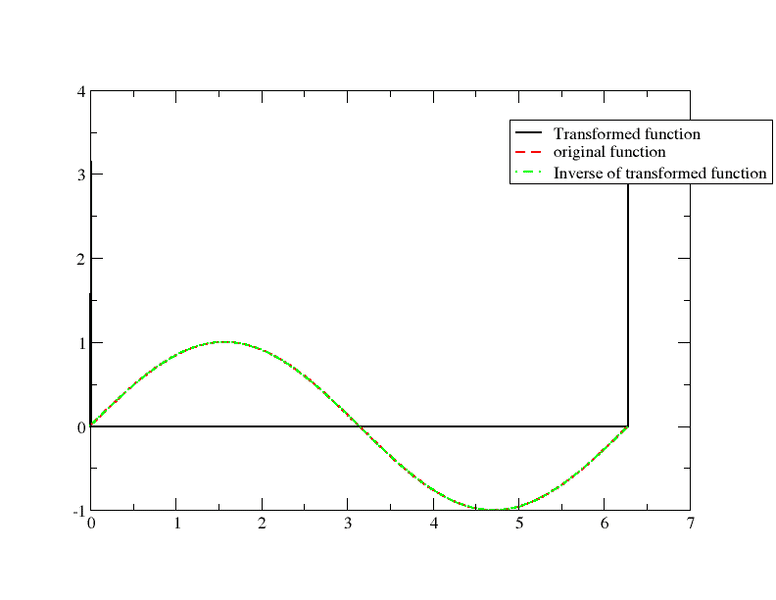I am using for the discrete case: ##x=i*\Delta x##, with i from 0 to N, and similarly ##k=i\Delta x##. Is my choice for the discrete k wrong? and how should I fix this?

#### Attachments

I think I've fixed it by taking ##\Delta k=\frac{2\pi}{L}##, where L is the length of the interval. Now, I was trying to represent a function using this Fourier transform, and the difficulty I encounter now is that I have a real function expressed as a set of real numbers and complex exponential. Is there anyway to visualize this as a real function?

It looks like you are using k as the frequency of sine, and also using k as the index of the summation?

•Mausam and Telemachus
Yes, what is wrong with that?

You see nothing wrong with ##\hat{f}_k = \sum_{j=0}^{N-1} e^{-ikx}\sin(kx)## in the context of the problem you were talking about in the original post?

Sorry, I don't see what you mean. Perhaps I'm committing the same mistake in this problem.

I have tried to expand a function in a Fourier series: I have ##f(x)=e^{\sin(x)}(\sin(x)^2+\sin(x))##.

I have computed the Fourier coefficients ##f_k## using the fftw in fortran. Then I approximate ##\displaystyle f(x_j) \sim \frac{1}{N} \sum_{k=0}^{N-1}f_k e^{ikx_j}## where ##x_j=j\frac{2\pi}{N}##. Where N is the number of points in the grid. This is the result I obtain for N=64: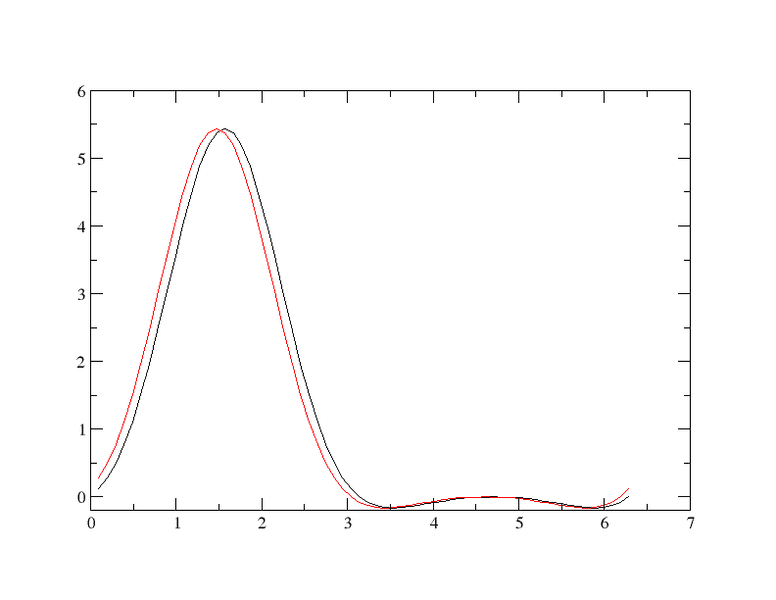If I increase N, I obtain a better representation.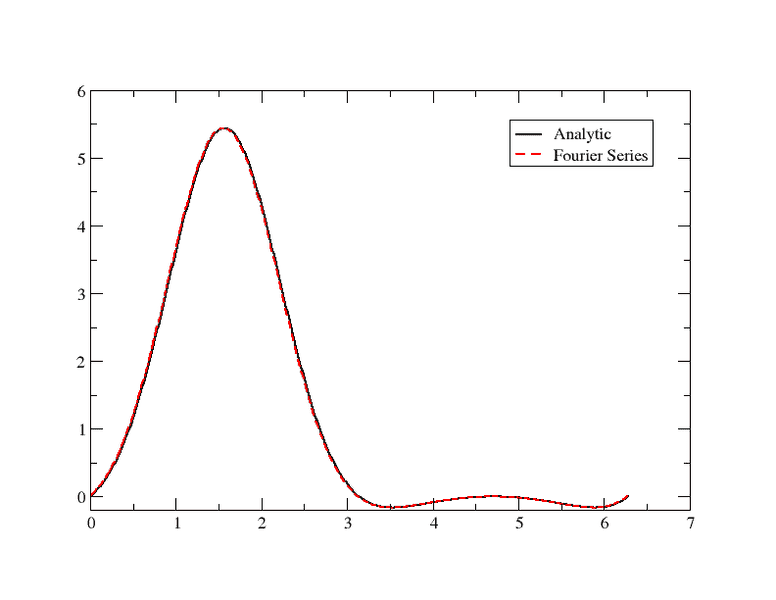However, due to the fact that the function is smooth, I hoped to have much better convergence than what I do.

I also have an analytic solution of a differential equation:
##u(x)-u''(x)=f(x)##
With periodic boundary conditions: ##u(x+2\pi)=u(x)##

Using a Fourier expansion ##\displaystyle f(x)=e^{\sin(x)}(\sin(x)^2+\sin(x))=\sum_{k=-\infty}^{\infty}\hat f_k e^{ikx}##, and similarly for ##\displaystyle u(x)=\sum_{k=-\infty}^{\infty}\hat u_k e^{ikx}##

I arrived to: ##\displaystyle u(x)=\sum_{k=-\infty}^{\infty}\frac{1}{1+k^2}\hat f_k e^{ikx}##.

In the discrete approximation I've used:
##\displaystyle u(x_j)\sim \frac{1}{N}\sum_{k=0}^{N-1}\frac{1}{1+k^2}\hat f_k e^{ikx_j}, \, x_j=j\frac{2\pi}{N}## (1)

Now there is some problem, and I don't know what it is. The analytic solution is: ##u(x)=e^{sin(x)}##, here is a plot of both, the analytic, and the one obtained by the Fourier series (1):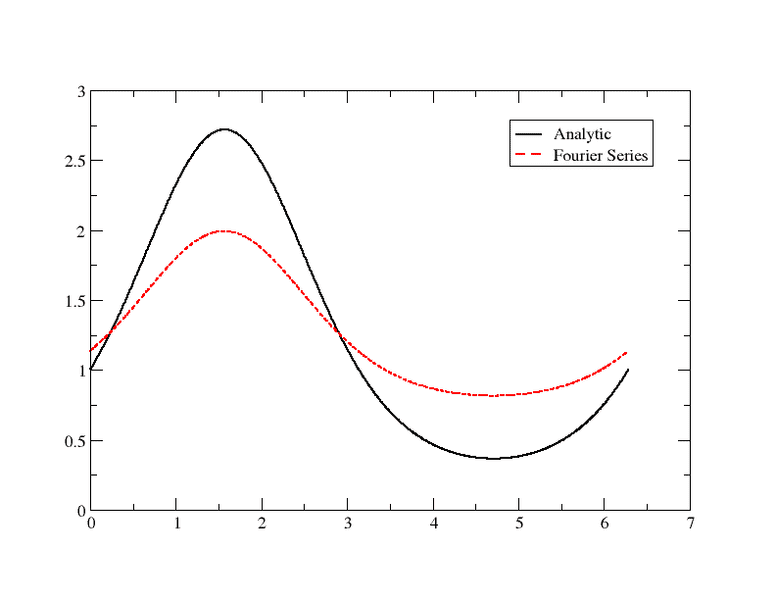the problem is possibly in the summation, as the solution is purely real, but if I plot the imaginary part for the resultant of the summation (1) I get: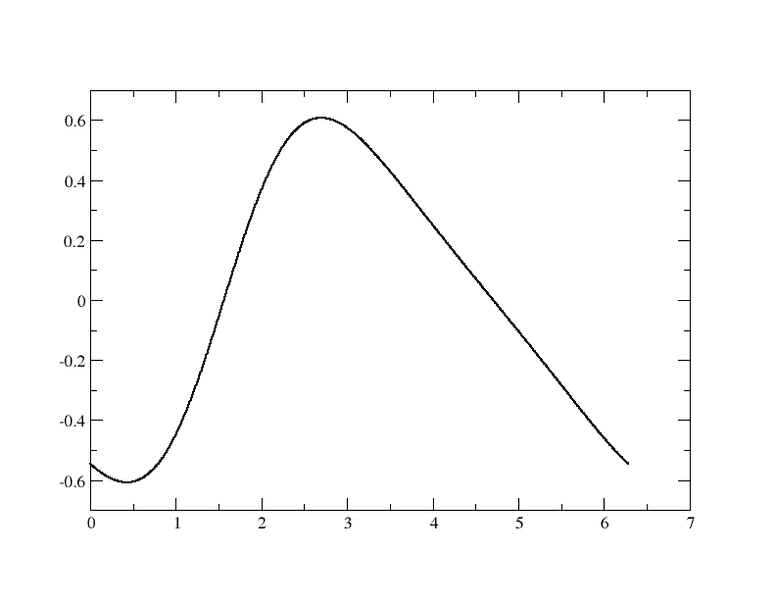I have also tried to run with k from ##-N/2## to ##N/2##, but I get zero.

This is the loop I use for the last problem:

Fortran:
     do j=1,N
x = j*dx
kv=zero
do i=1,N
kn=i-1
ct=one/(N*(one+kn**2))
kv=ct*zexp(im*kn*x)*zout(i)+kv
enddo
user = dreal(kv)
cuser = dimag(kv)
write(16,*) x,' ',user
write(18,*) x,' ',cuser
enddo
zout(i) are the Fourier coefficients obtained by fftw, and I am is the imaginary unit.

I don't know if this is related to the problem you've both mentioned before, or if this has something to do with what is explained in here with respect to the ordering of the Fourier coefficients: http://www.fftw.org/fftw3_doc/The-1d-Discrete-Fourier-Transform_0028DFT_0029.html

Note also that we use the standard “in-order” output ordering—the k-th output corresponds to the frequency k/n (or k/T, where T is your total sampling period). For those who like to think in terms of positive and negative frequencies, this means that the positive frequencies are stored in the first half of the output and the negative frequencies are stored in backwards order in the second half of the output. (The frequency -k/n is the same as the frequency (n-k)/n.)

#### Attachments

Last edited:
You see nothing wrong with ##\hat{f}_k = \sum_{j=0}^{N-1} e^{-ikx}\sin(kx)## in the context of the problem you were talking about in the original post?
Is this related to the fact that the function is ##2\pi## periodic? that gives a bound in k, and actually set it to be a discrete set even for continuous x, is that it?

No, it's that the ##k## in ##\sin kx## isn't the same ##k## as the ##k## in ##e^{-ikx}##.

•Telemachus
I wasn't aware of that. From Euler Formula I have:

##\sin(kx)=\frac{e^{ikx}-e^{-ikx}}{2i}##, So, when I do the projection I see that I will have: ##\hat f_k=\sum_{j=0}^{N-1}e^{-ik}\frac{e^{ikx}-e^{-ikx}}{2i}##
so the k in the exponential is twice the k in the sine function?

In the original post, you were looking at a function ##f(x) = \sin kx## with the specific case where ##k=2##. In your expression for ##\hat{f}_k##, you said ##k=0,1,2,\dots,N-1##. And then in your expression for ##f_j##, ##k## is the summation index. Using the same symbol to mean different things is confusing at best. More likely, it's going to lead to a mistake.

•Telemachus
In the original post, you were looking at a function ##f(x) = \sin kx## with the specific case where ##k=2##. In your expression for ##\hat{f}_k##, you said ##k=0,1,2,\dots,N-1##. And then in your expression for ##f_j##, ##k## is the summation index. Using the same symbol to mean different things is confusing at best. More likely, it's going to lead to a mistake.

Yes, you are right, I was actually seeking for the wrong k in the transformed function. I didn't thought of it, thank you. Should I create a new thread for the question in post #6? I didn't do it because it is about discrete Fourier transforms too.

No, I don't think that's necessary. Post 6 provides some context for what you were asking originally.

For what it's worth, I tried the calculation you did (in post 6) in Mathematica and got the results you were expecting. Because of rounding errors, the values of ##u(x_j)## had tiny imaginary parts, but ignoring those and plotting just the real part appears to have reproduced the analytical solution. I used values of ##k## running from ##-N/2## to ##N/2-1##.

It's probably a good idea to make sure your transformations are working for simpler cases, as you seem to be trying now, to pinpoint where your problem lies.

•Telemachus
Yes, that gives me zero. I'll post the whole code in case that anyone wants to try. I'm using a unix system, and compile with gfortran.

To compile I use:
gfortran program.for -L/usr/local/lib -lfftw3 -lm -o program.x

Fortran:
    program fourser
c...FFTW transformation
double complex zin, zout
parameter (nmax=2**10)
dimension zin(nmax), zout(nmax)
integer*8 plan
double complex zero,zone,eye,kv
real*8 rzero,one
real*8 rmin,rmax,dx
integer N,kn
real*8 x,ct,kr,user,u
data rzero,one,two/0.0d0,1.0d0,2.0d0/
include "/usr/local/include/fftw3.f"
zero = dcmplx(rzero,rzero)
eye = dcmplx(rzero,one)
zone = dcmplx(one,rzero)
Pi = two*dasin(one)
rmin = rzero
rmax = two*Pi
N = 1000
dx = (rmax-rmin)/N
c... output files
open(unit=15,file='fx2.dat',status='unknown')
open(unit=16,file='user2.dat',status='unknown')
open(unit=17,file='uan2.dat',status='unknown')
open(unit=18,file='cuser2.dat',status='unknown')
c   open(unit=25,file='Invfk.dat',status='unknown')
do i=1,N
x = i*dx
zin(i) = dexp(dsin(x))*(dsin(x)**2+dsin(x))
rz = dreal(zin(i))
ri = dimag(zin(i))
ra = cdabs(zin(i))
u = dexp(dsin(x))
write(15,*) x,' ',rz
write(17,*) x,' ',u
enddo
c... Direct Fast Fourier Transform
call dfftw_plan_dft_1d(plan,N,zin,zout,
+                        FFTW_FORWARD,FFTW_ESTIMATE)
call dfftw_execute(plan)
call dfftw_destroy_plan(plan)
c... Solve problem
do j=1,N
x = j*dx
kv=zero
do i=1,N
kn=-N/2+1-i
ct=one/(N*(one+kn**2))
kv=ct*zexp(eye*kn*x)*zout(i)+kv
enddo
user = dreal(kv)
cuser = dimag(kv)
write(16,*) x,' ',user
write(18,*) x,' ',cuser
enddo
close(15)
close(16)
close(17)
close(18)
stop
end

I think that possible the problem is in how the coefficients are ordered, but I think I have tried all the possibilities.

Last edited:
Rather than doing the summation for the inverse DFT yourself, why not let FFTW do it? You just need one loop to calculate ##\hat{u}_k## and the send the resulting array to FFTW.

•Telemachus
Oh, I'll try that, its a good idea.

Yes, that was a good idea. Now I have some constant messing around, but the convergence seems good.

Sorry, after a check, I still having the complex component for the solution.

#### Attachments

Last edited:
When you calculate ##\hat{u}_k##, you're probably using the wrong value for ##k## in ##1/(1+k^2)##.

•Telemachus
Yes, that was it. Thanks!

Sorry, I saw the stupid thing I did with the k the other day and I laughed. Anyway, of course in the program was well set.

•Laurence Bowes